# Solar core

Updated on
Covid-19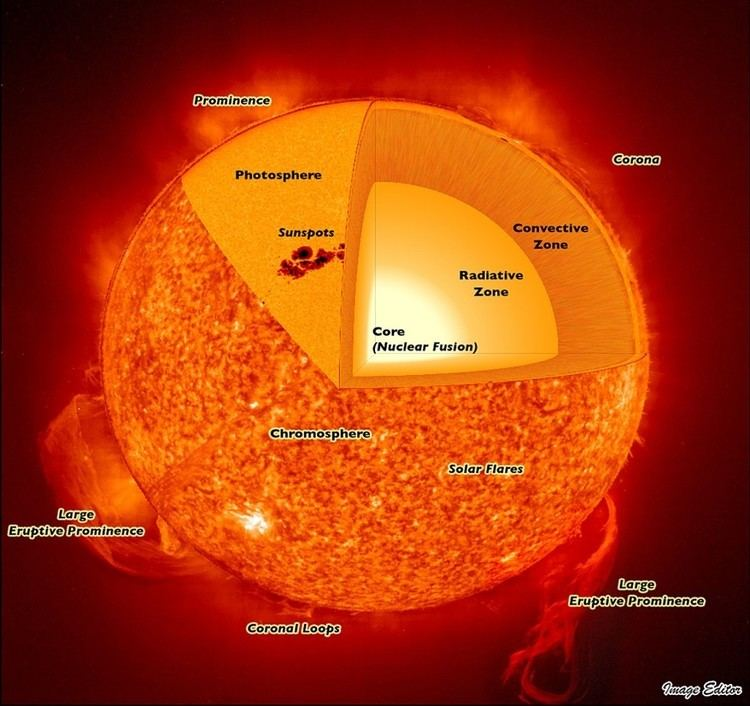The core of the Sun is considered to extend from the center to about 0.2 to 0.25 of solar radius. It is the hottest part of the Sun and of the Solar System. It has a density of 150 g/cm³ (150 times the density of liquid water) at the center, and a temperature of 15 million degrees Celsius. The core is made of hot, dense gas in the plasmic state (ions and electrons), at a pressure estimated at 265 billion bar (3.84 trillion psi or 26.5 petapascals (PPa)) at the center. Due to fusion, the composition of the solar plasma drops from 68-70% hydrogen by mass at the outer core, to 33% hydrogen at the core/Sun center.

## Contents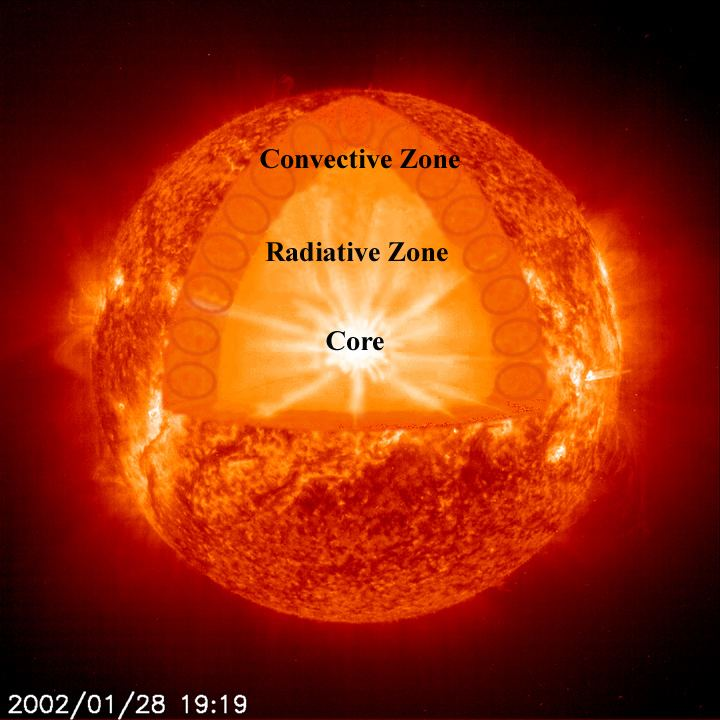The core inside 0.20 of the solar radius, contains 34% of the Sun's mass, but only 0.8% of the Sun's volume. Inside 0.24 solar radius, the core generates 99% of the fusion power of the Sun. There are two distinct reactions in which four hydrogen nuclei may eventually result in one helium nucleus: the proton-proton chain reaction – which is responsible for most of the Sun's released energy – and the CNO cycle.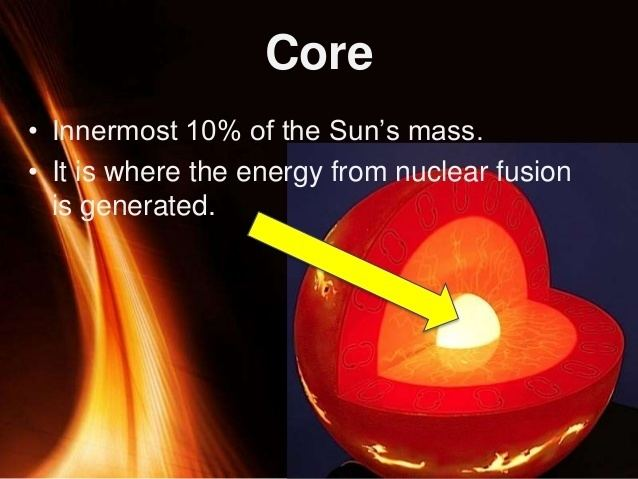## Composition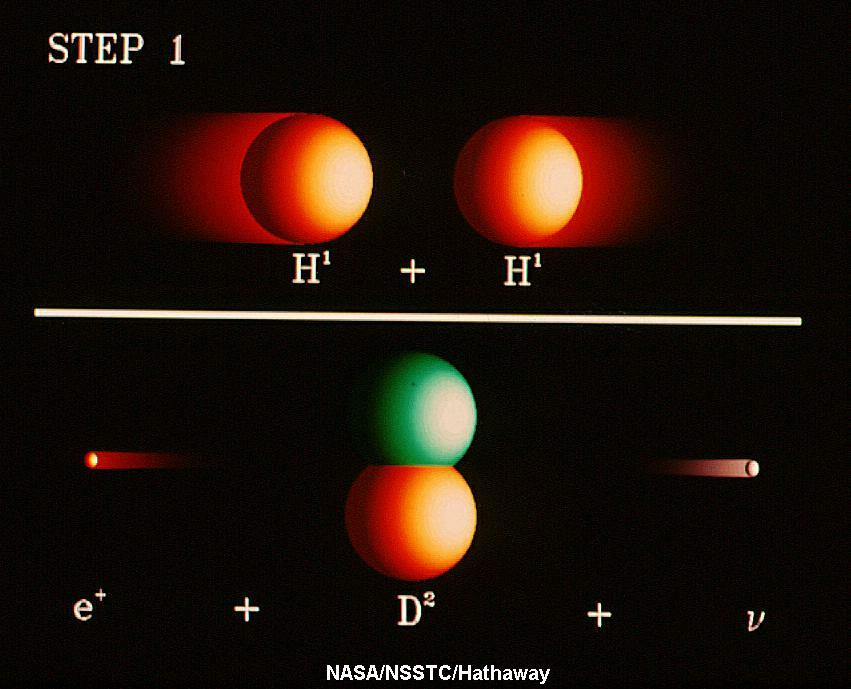The Sun at the photosphere is about 73-74% by mass hydrogen, which is the same composition as the atmosphere of Jupiter, and the primordial composition of hydrogen and helium at the earliest star formation after the Big Bang. However, as depth into the Sun increases, fusion decreases the fraction of hydrogen. Traveling inward, hydrogen mass fraction starts to decrease rapidly after the core radius has been reached (it is still about 70% at a radius 25% of the Sun's radius) and inside this, the hydrogen fraction drops rapidly as the core is traversed, until it reaches a low of about 33% hydrogen, at the Sun's center (radius zero). All but 2% of the remaining plasma mass (i.e., 65%) is helium, at the center of the Sun.

## Energy conversion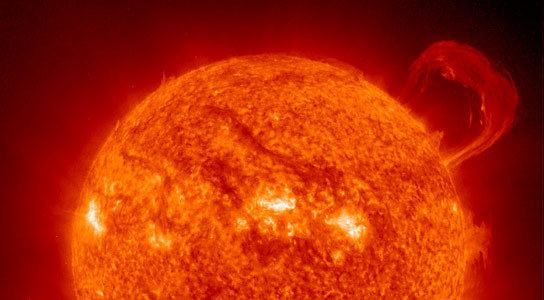Approximately 3.6×1038 protons (hydrogen nuclei), or roughly 299 million metric tons of hydrogen, are converted into helium nuclei every second releasing energy at a rate of 3.86×1026 joules per second.The core produces almost all of the Sun's heat via fusion: the rest of the star is heated by the outward transfer of heat from the core. The energy produced by fusion in the core, except a small part carried out by neutrinos, must travel through many successive layers to the solar photosphere before it escapes into space as sunlight or kinetic energy of particles. The energy conversion per unit time (power) of fusion in the core varies with distance from the solar center. At the center of the Sun, fusion power is estimated by models to be about 276.5 watts/m3. Despite its intense temperature, the peak power generating density of the core overall is similar to an active compost heap, and is lower than the power density produced by the metabolism of an adult human. The Sun is much hotter than a compost heap due to the Sun's enormous volume.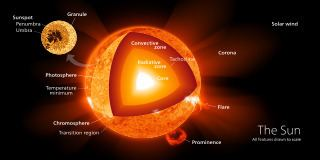The low power outputs occurring inside the fusion core of the Sun may also be surprising, considering the large power which might be predicted by a simple application of the Stefan–Boltzmann law for temperatures of 10 to 15 million kelvin. However, layers of the Sun are radiating to outer layers only slightly lower in temperature, and it is this difference in radiation powers between layers which determines net power generation and transfer in the solar core.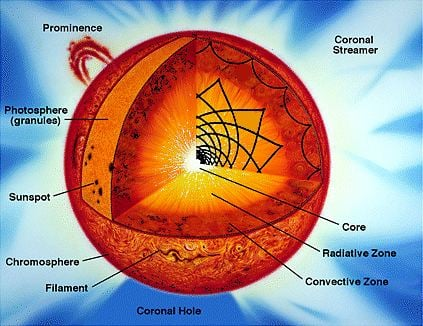At 19% of the solar radius, near the edge of the core, temperatures are about 10 million kelvin and fusion power density is 6.9 W/m3, which is about 2.5% of the maximum value at the solar center. The density here is about 40 g/cm3, or about 27% of that at the center. Some 91% of the solar energy is produced within this radius. Within 24% of the radius (the outer "core" by some definitions), 99% of the Sun's power is produced. Beyond 30% of the solar radius, where temperature is 7 million K and density has fallen to 10 g/cm3 the rate of fusion is almost nil. There are two distinct reactions in which 4 H nuclei may eventually result in one He nucleus: "proton-proton chain reaction" and the "CNO cycle" (see below).

## Proton-proton chain reaction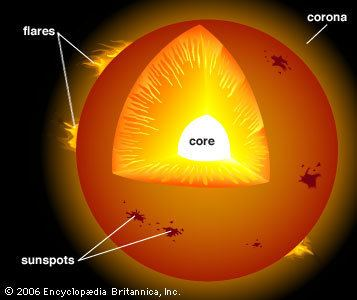The first reaction in which 4 H nuclei may eventually result in one He nucleus is known as the proton-proton chain, is: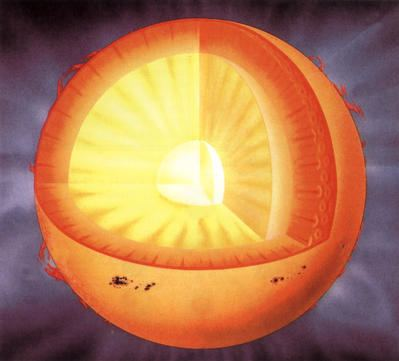{ 1 H + 1 H 2 D + e + + v then 2 D + 1 H 3 H e + γ then 3 H e + 3 H e 4 H e + 1 H + 1 H

This reaction sequence is thought to be the most important one in the solar core. The characteristic time for the first reaction is about one billion years even at the high densities and temperatures of the core, due to the necessity for the weak force to cause beta decay before the nucleons can adhere (which rarely happens in the time they tunnel toward each other, to be close enough to do so). The time that deuterium and helium-3 in the next reactions last, by contrast, are only about 4 seconds and 400 years. These later reactions proceed via the nuclear force and are thus much faster. The total energy released by these reactions in turning 4 hydrogen atoms into 1 helium atom is 26.7 MeV.

## CNO cycle

The second reaction sequence, in which 4 H nuclei may eventually result in one He nucleus is called the carbon-nitrogen-oxygen cycle – or "CNO cycle" for short – generates less than 10% of the total solar energy. This involves carbon atoms which are not consumed in the overall process. The details of this "carbon cycle" are as follows:

{ 12 C + 1 H 13 N + γ then 13 N 13 C + e + + ν then 13 C + 1 H 14 N + γ then 14 N + 1 H 15 O + γ then 15 O 15 N + e + + ν then 15 N + 1 H 12 C + 4 H e + γ

This process can be further understood by the picture on the right, starting from the top in clockwise direction.

## Equilibrium

The rate of nuclear fusion depends strongly on density. Therefore, the fusion rate in the core is in a self-correcting equilibrium: a slightly higher rate of fusion would cause the core to heat up more and expand slightly against the weight of the outer layers. This would reduce the fusion rate and correct the perturbation; and a slightly lower rate would cause the core to cool and shrink slightly, increasing the fusion rate and again reverting it to its present level.

However the Sun gradually becomes hotter during its time on the main sequence, because the helium atoms in the core are heavier than the hydrogen atoms they were fused from. This increases the gravitational pressure on the core which is resisted by a gradual increase in the rate at which fusion occurs. This process speeds up over time as the core gradually becomes heavier. It is estimated that the sun has become 30% brighter in the last four and a half billion years and will continue to increase in brightness by 1% every 100 million years.

## Energy transfer

The high-energy photons (gamma rays) released in fusion reactions take indirect paths to the Sun's surface. According to current models, random scattering from free electrons in the solar radiative zone (the zone within 75% of the solar radius, where heat transfer is by radiation) sets the photon diffusion time scale (or "photon travel time") from the core to the outer edge of the radiative zone at about 170,000 years. From there they cross into the convective zone (the remaining 25% of distance from the Sun's center), where the dominant transfer process changes to convection, and the speed at which heat moves outward becomes considerably faster.

In the process of heat transfer from core to photosphere, each gamma ray in the Sun's core is converted during scattering into several million visible light photons before escaping into space. Neutrinos are also released by the fusion reactions in the core, but unlike photons they very rarely interact with matter, so almost all are able to escape the Sun immediately. For many years measurements of the number of neutrinos produced in the Sun were much lower than theories predicted, a problem which was recently resolved through a better understanding of neutrino oscillation.

## References

Similar Topics
Terry Casey
Peter Francese
Danny Jones (rugby league)
Topics